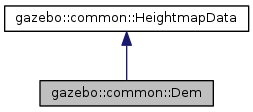##gazebo::common::Dem Class Reference

`#include <Dem.hh>`

Inheritance diagram for gazebo::common::Dem:[legend]
Collaboration diagram for gazebo::common::Dem:[legend]

## Public Member Functions

Dem ()
Constructor. More...

virtual ~Dem ()
Destructor. More...

void FillHeightMap (int _subSampling, unsigned int _vertSize, const math::Vector3 &_size, const math::Vector3 &_scale, bool _flipY, std::vector< float > &_heights)
Create a lookup table of the terrain's height. More...

double GetElevation (double _x, double _y)
Get the elevation of a terrain's point in meters. More...

void GetGeoReferenceOrigin (math::Angle &_latitude, math::Angle &_longitude)
Get the georeferenced coordinates (lat, long) of the terrain's origin in WGS84. More...

unsigned int GetHeight () const
Get the terrain's height. More...

float GetMaxElevation () const
Get the terrain's maximum elevation in meters. More...

float GetMinElevation () const
Get the terrain's minimum elevation in meters. More...

unsigned int GetWidth () const
Get the terrain's width. More...

double GetWorldHeight () const
Get the real world height in meters. More...

double GetWorldWidth () const
Get the real world width in meters. More...Public Member Functions inherited from gazebo::common::HeightmapData
virtual ~HeightmapData ()
Destructor. More...

## Constructor & Destructor Documentation

 gazebo::common::Dem::Dem ( )

Constructor.

 virtual gazebo::common::Dem::~Dem ( )
virtual

Destructor.

## Member Function Documentation

 void gazebo::common::Dem::FillHeightMap ( int _subSampling, unsigned int _vertSize, const math::Vector3 & _size, const math::Vector3 & _scale, bool _flipY, std::vector< float > & _heights )
virtual

Create a lookup table of the terrain's height.

Parameters
 [in] _subsampling Multiplier used to increase the resolution. Ex: A subsampling of 2 in a terrain of 129x129 means that the height vector will be 257 * 257. [in] _vertSize Number of points per row. [in] _size Real dimmensions of the terrain in meters. [in] _scale Vector3 used to scale the height. [in] _flipY If true, it inverts the order in which the vector is filled. [out] _heights Vector containing the terrain heights.

Implements gazebo::common::HeightmapData.

 double gazebo::common::Dem::GetElevation ( double _x, double _y )

Get the elevation of a terrain's point in meters.

Parameters
 [in] _x X coordinate of the terrain. [in] _y Y coordinate of the terrain.
Returns
Terrain's elevation at (x,y) in meters.
 void gazebo::common::Dem::GetGeoReferenceOrigin ( math::Angle & _latitude, math::Angle & _longitude )

Get the georeferenced coordinates (lat, long) of the terrain's origin in WGS84.

Parameters
 [out] _latitude Georeferenced latitude. [out] _longitude Georeferenced longitude.
 unsigned int gazebo::common::Dem::GetHeight ( ) const
virtual

Get the terrain's height.

Due to the Ogre constrains, this value will be a power of two plus one. The value returned might be different that the original DEM height because GetData() adds the padding if necessary.

Returns
The terrain's height (points) satisfying the ogre constrains (squared terrain with a height value that must be a power of two plus one).

Implements gazebo::common::HeightmapData.

 float gazebo::common::Dem::GetMaxElevation ( ) const
virtual

Get the terrain's maximum elevation in meters.

Returns
The maximum elevation (meters).

Implements gazebo::common::HeightmapData.

 float gazebo::common::Dem::GetMinElevation ( ) const

Get the terrain's minimum elevation in meters.

Returns
The minimum elevation (meters).
 unsigned int gazebo::common::Dem::GetWidth ( ) const
virtual

Get the terrain's width.

Due to the Ogre constrains, this value will be a power of two plus one. The value returned might be different that the original DEM width because GetData() adds the padding if necessary.

Returns
The terrain's width (points) satisfying the ogre constrains (squared terrain with a width value that must be a power of two plus one).

Implements gazebo::common::HeightmapData.

 double gazebo::common::Dem::GetWorldHeight ( ) const

Get the real world height in meters.

Returns
Terrain's real world height in meters.
 double gazebo::common::Dem::GetWorldWidth ( ) const

Get the real world width in meters.

Returns
Terrain's real world width in meters.
 int gazebo::common::Dem::Load ( const std::string & _filename = `""` )